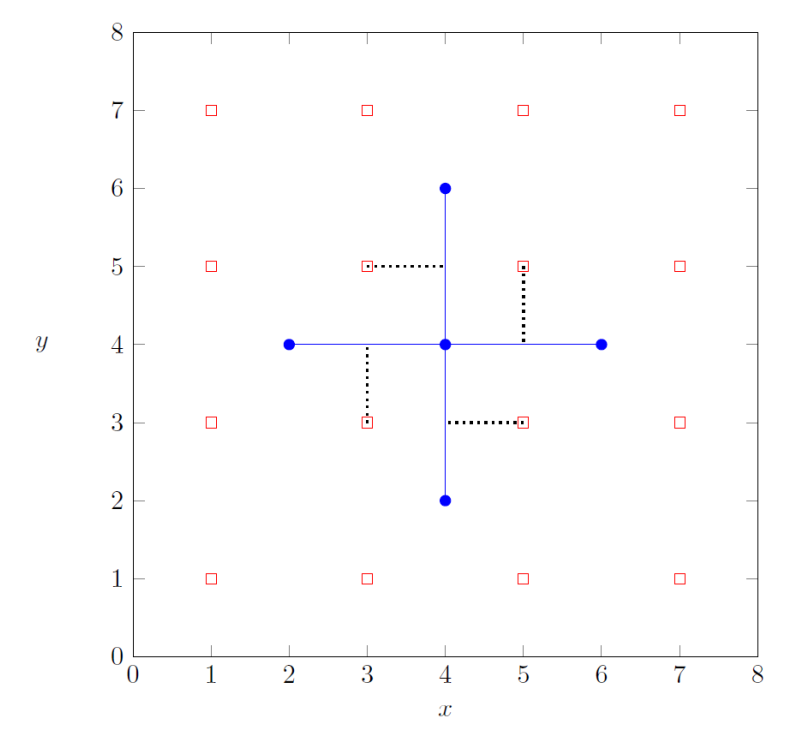# #662. 【IOI2021】parks

### 实现细节

int construct_roads(int[] x, int[] y)
• $x, y$：长度为 $n$ 的两个数组。对所有 $i$（$0 \le i \le n - 1$），喷泉 $i$ 是一个点 $(x[i], y[i])$，这里 $x[i]$ 和 $y[i]$ 都是偶数。
• 如果存在某个建设方案，函数应当调用 build（参见下文）恰好一次来报告建设方案，并紧接着返回 $1$。
• 否则，函数应当返回 $0$，并且不做 build 的任何调用。
• 该函数将被调用恰好一次。

void build(int[] u, int[] v, int[] a, int[] b)

• $u, v$：长度为 $m$ 的两个数组，表示要建造的道路。这些道路的编号为从 $0$ 到 $m - 1$。对所有的 $j$（$0 \le j \le m - 1$），道路 $j$ 要连接喷泉 $u[j]$ 和 $v[j]$。每条道路必须是长度为 $2$ 的横向或纵向线段。任意两条不同的道路，最多只能有一个公共端点（某个喷泉）。这些道路在建成之后，必须能够沿着它们就可以在任意两个喷泉之间互相抵达。
• $a, b$：长度为 $m$ 的两个数组，表示长椅。对所有的 $j$（$0 \le j \le m - 1$），将在 $(a[j], b[j])$ 处摆放一个长椅，并且分配给道路 $j$。不同的长椅不能摆放在同一位置。

### 输入格式

• 第 $1$ 行：$n$
• 第 $2 + i$ 行（$0 \le i \le n - 1$）：$x[i] \; y[i]$

### 输出格式

• 第 $1$ 行：construct_roads 的返回值

• 第 $2$ 行：$m$
• 第 $3 + j$ 行（$0 \le j \le m - 1$）：$u[j] \; v[j] \; a[j] \; b[j]$

### 样例一

#### input

5
4 4
4 6
6 4
4 2
2 4



#### output

1
4
0 2 5 5
0 1 3 5
3 0 5 3
4 0 3 3



#### explanation

construct_roads([4, 4, 6, 4, 2], [4, 6, 4, 2, 4])

• 喷泉 $0$ 坐落在 $(4, 4)$ 处，
• 喷泉 $1$ 坐落在 $(4, 6)$ 处，
• 喷泉 $2$ 坐落在 $(6, 4)$ 处，
• 喷泉 $3$ 坐落在 $(4, 2)$ 处，
• 喷泉 $4$ 坐落在 $(2, 4)$ 处。

$0$ $0, 2$ $(5, 5)$
$1$ $0, 1$ $(3, 5)$
$2$ $3, 0$ $(5, 3)$
$3$ $4, 0$ $(3, 3)$build([0, 0, 3, 4], [2, 1, 0, 0], [5, 3, 5, 3], [5, 5, 3, 3])

### 样例二

#### input

2
2 2
4 6



#### output

0



#### explanation

construct_roads([2, 4], [2, 6])

### 数据范围

• $1 \le n \le 200 \, 000$
• $2 \le x[i], y[i] \le 200 \, 000$（对于所有 $0 \le i \le n - 1$）
• $x[i]$ 和 $y[i]$ 都是偶数（对于所有 $0 \le i \le n - 1$）
• 任意两座喷泉的位置均不相同

$1$ $5$ $x[i] = 2$（对于所有 $0 \le i \le n - 1$）
$2$ $10$ $2 \le x[i] \le 4$（对于所有 $0 \le i \le n - 1$）
$3$ $15$ $2 \le x[i] \le 6$（对于所有 $0 \le i \le n - 1$）
$4$ $20$ 至多只有一种道路建设方案，能够让游客在任意两座喷泉之间沿着这些道路即可互相抵达
$5$ $20$ 任意四座喷泉都不会构成某一个 $2 \times 2$ 正方形的四个顶点
$6$ $30$ 没有额外的约束条件